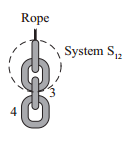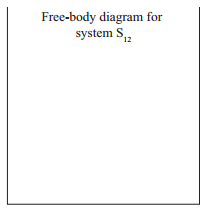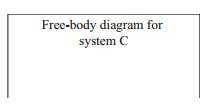# Problem: Let S12 represent the system consisting of links 1 and 2 of the chain in problem 2 (i.e., treat links 1 and 2 as a single object).a. In the space at right, draw and label a free-body diagram for system S12.  b. Compare the forces that appear on your free-body diagram for system S12 to those that appear on your diagrams for links 1 and 2 in problem 2.i. For each of the forces that appear on your diagram for system S12, list the corresponding force (or forces) on your diagrams for links 1 and 2ii. Are there any forces on your diagrams for links 1 and 2 that you did not list? If so, what characteristics do these forces have in common that none of the others share?c. Let C represent the system consisting of the whole chain. Draw and label a free-body diagram for C. Make sure that your diagram is consistent with the reasoning you used in part b.

###### FREE Expert Solution

In this problem, we'll identify the forces acting on an object and the relationship between the forces.

a.

We'll use "u" for upward forces and "d" for downward forces for the forces between links

The forces on the system are:

-The rope pulls the system upward (T)

-Link three exerts a downward force on the system (F3d)

99% (154 ratings)###### Problem Details

Let S12 represent the system consisting of links 1 and 2 of the chain in problem 2 (i.e., treat links 1 and 2 as a single object).a. In the space at right, draw and label a free-body diagram for system S12.b. Compare the forces that appear on your free-body diagram for system S12 to those that appear on your diagrams for links 1 and 2 in problem 2.
i. For each of the forces that appear on your diagram for system S12, list the corresponding force (or forces) on your diagrams for links 1 and 2
ii. Are there any forces on your diagrams for links 1 and 2 that you did not list? If so, what characteristics do these forces have in common that none of the others share?
c. Let C represent the system consisting of the whole chain. Draw and label a free-body diagram for C. Make sure that your diagram is consistent with the reasoning you used in part b.Frequently Asked Questions

What scientific concept do you need to know in order to solve this problem?

Our tutors have indicated that to solve this problem you will need to apply the Forces in Connected Systems of Objects concept. You can view video lessons to learn Forces in Connected Systems of Objects. Or if you need more Forces in Connected Systems of Objects practice, you can also practice Forces in Connected Systems of Objects practice problems.